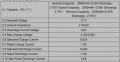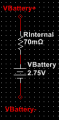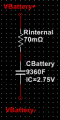# Need Battery 18650 SPICE Model for MultiSim

#### Murray Chen

Joined Jun 12, 2019
11
I tried googling but couldn't find anything about Battery 18650 SPICE Model for MultiSim.
Does any one who can help me on this?
Thanks.

#### Audioguru

Joined Dec 20, 2007
11,249
A battery is a simple DC voltage source. Every Sim program has a simple DC voltage source.
Go to www.batteryuniversity.com to learn the fully charged voltage (4.2V), the average voltage during a discharge (3.7V) and the minimum allowed voltage (3.2V) of an 18650 battery cell.

•JohnInTX

#### crutschow

Joined Mar 14, 2008
27,945
If you want to simulate the charging/discharging of a battery, then a large capacitor (several Farads or more depending upon the battery capacity) can help simulate that.

#### MrAl

Joined Jun 17, 2014
8,504
Hi,

It depends what you are interested in simulating.

If you just want to do a general check of your charge circuit, then use a voltage source with a small resistor in series. 0.05 ohms would be somewhat typical.

If you want to check charge time, then use a large value capacitor with small resistor like 0.05 Ohms in series.
If i remember right, the value of the cap should be 3600 Farads per Ampere Hour battery capacity with the initial voltage value set to the min cell voltage before a charge.

There are really better models on the web but you probably dont want to go through the trouble of setting all the parameters.

#### Murray Chen

Joined Jun 12, 2019
11
Hi,

It depends what you are interested in simulating.

If you just want to do a general check of your charge circuit, then use a voltage source with a small resistor in series. 0.05 ohms would be somewhat typical.

If you want to check charge time, then use a large value capacitor with small resistor like 0.05 Ohms in series.
If i remember right, the value of the cap should be 3600 Farads per Ampere Hour battery capacity with the initial voltage value set to the min cell voltage before a charge.

There are really better models on the web but you probably dont want to go through the trouble of setting all the parameters.

Hi,

My Lithium-Ion Battery has specifications as follows,For check of charge circuit, I choose series resistor 70m Ohms for the worst case with initial voltage source 2.75V,To check charge time, I use the plan shown below (Battery capacity 9360F = 3600*2600*10^(-3), with the initial voltage value 2.75V),With such kind of arrangements, does it meet your comments or not?

By the way, is there any better model's URL could be provided for my reference?

Thanks.

#### Alec_t

Joined Sep 17, 2013
12,260
Unless you need to model some unusual property of a battery you might as well use a simple battery model as suggested above rather than a super-accurate 'better' one. The more complex the model the longer the sim takes to run.

#### MrAl

Joined Jun 17, 2014
8,504
Hello again,

I went back over the idea and found that the cap value is better placed at:
C=3600*AH/1.4

which means:

The whole idea is that the cap must charge from 2.75v to 4.15v (or a little less) in a bit less than 2.6 hours with a constant current of 1 amp.
dv/dt=i/C
(4.15-2.75)/(2.6*3600)=1/C

and that is with 0.05 Ohms for the series R. With R=0.07 it would be closer to 6783 Farads but that's not too critical.

#### Murray Chen

Joined Jun 12, 2019
11
Hello again,

I went back over the idea and found that the cap value is better placed at:
C=3600*AH/1.4

which means:

The whole idea is that the cap must charge from 2.75v to 4.15v (or a little less) in a bit less than 2.6 hours with a constant current of 1 amp.
dv/dt=i/C
(4.15-2.75)/(2.6*3600)=1/C

and that is with 0.05 Ohms for the series R. With R=0.07 it would be closer to 6783 Farads but that's not too critical.
Hi,

I've 2 questions,
1. In "C=3600*AH/1.4", what the magic number 1.4 is?
2. In "(4.15-2.75)/(2.6*3600)=1/C", how the expression "2.6*3600" could get unit of time, i.e. seconds?

Thanks.

#### MrAl

Joined Jun 17, 2014
8,504
Hi,

I've 2 questions,
1. In "C=3600*AH/1.4", what the magic number 1.4 is?
2. In "(4.15-2.75)/(2.6*3600)=1/C", how the expression "2.6*3600" could get unit of time, i.e. seconds?

Thanks.
Hi,

They are both because the cap does not charge from zero to max voltage, it chargers from some value like 2.75v to 4.2v. That changes teh overall capacitance of the capacitor itself. The "1.4" happens to come from that.

For the second though we have:
V/Q=1/C
or taking the inverse we get usual:
Q/V=C

with charge A in Ampere Seconds and V in volts (voltage differential) and C in Farads.

The value of 4.15 volts is based on a charge current of 1 Ampere and series resistance of 0.050 Ohms. That is the transition point from constant current to constant voltage. This provides for an estimate of charge time and therefore the value of the equivalent capacitance. It's not that critical though.

#### Murray Chen

Joined Jun 12, 2019
11
Hi,

They are both because the cap does not charge from zero to max voltage, it chargers from some value like 2.75v to 4.2v. That changes teh overall capacitance of the capacitor itself. The "1.4" happens to come from that.

For the second though we have:
V/Q=1/C
or taking the inverse we get usual:
Q/V=C

with charge A in Ampere Seconds and V in volts (voltage differential) and C in Farads.

The value of 4.15 volts is based on a charge current of 1 Ampere and series resistance of 0.050 Ohms. That is the transition point from constant current to constant voltage. This provides for an estimate of charge time and therefore the value of the equivalent capacitance. It's not that critical though.
Hi,
Thanks for your detailed description. It's more clear now.